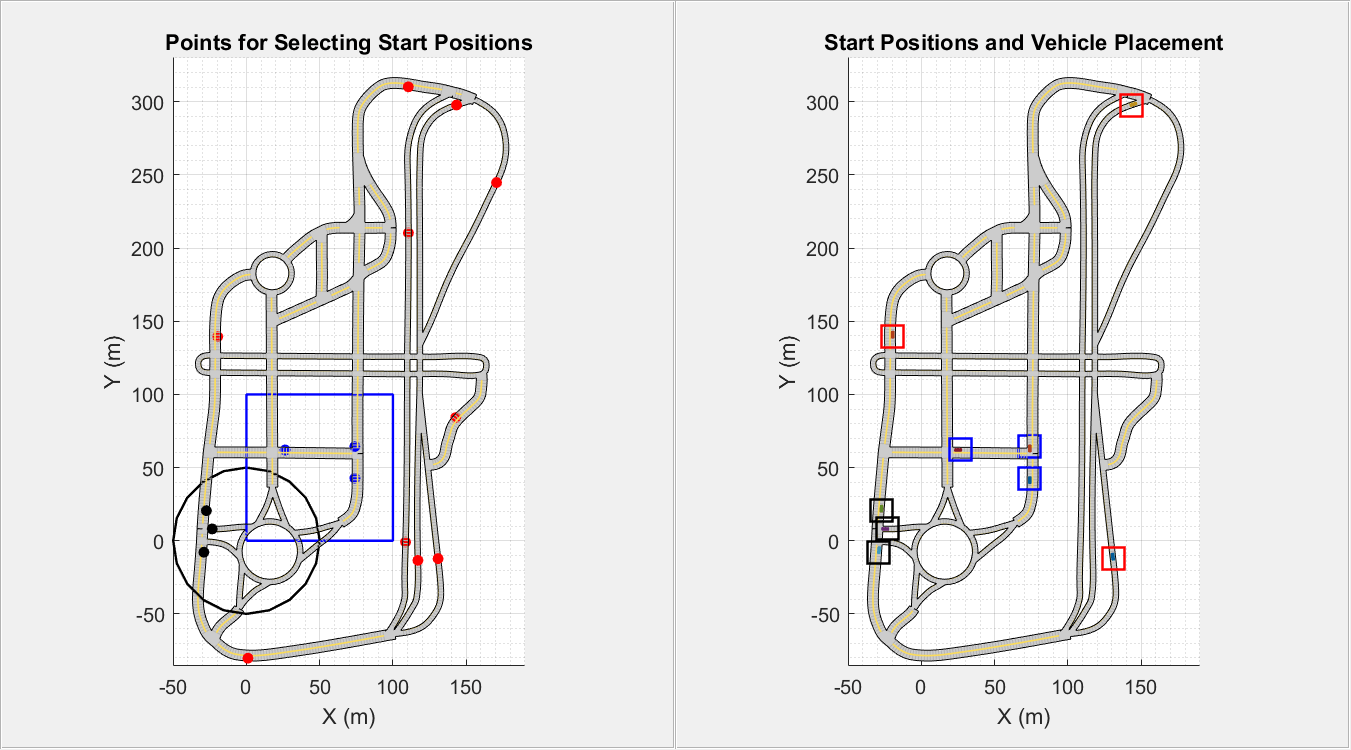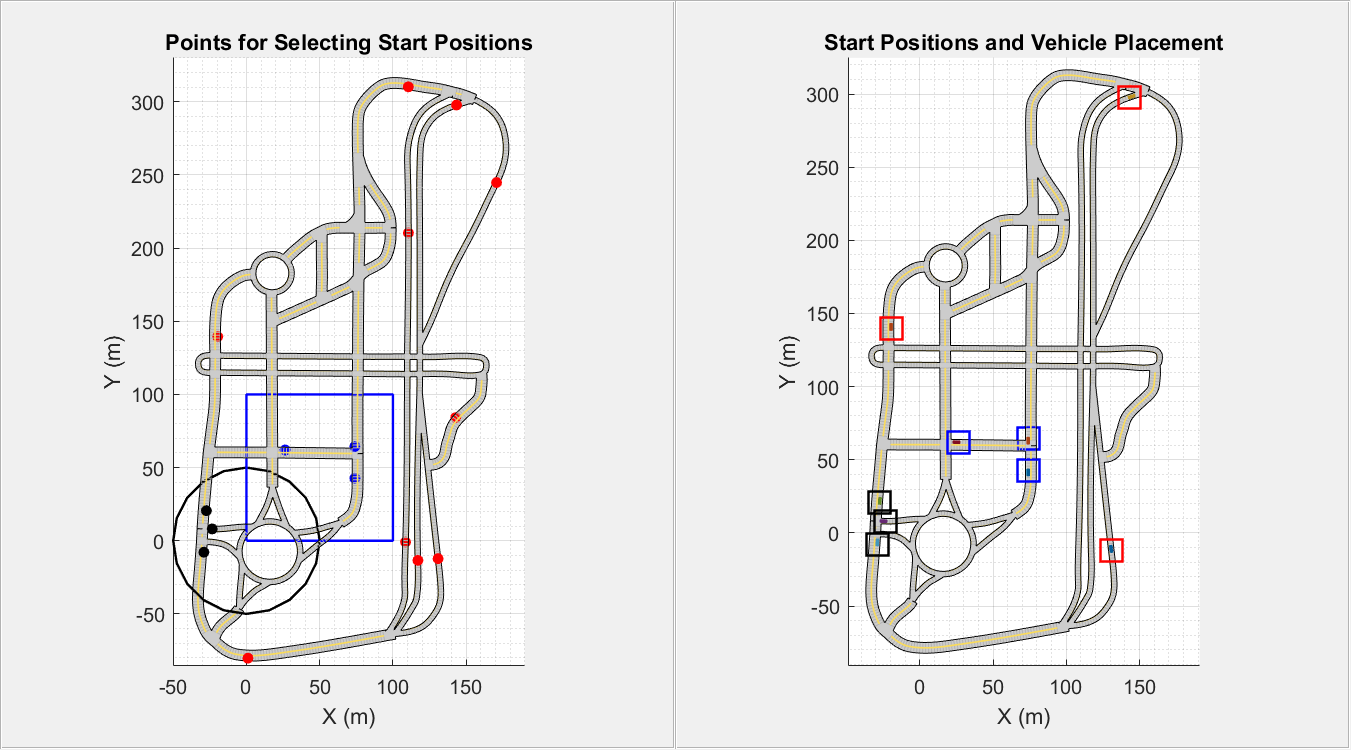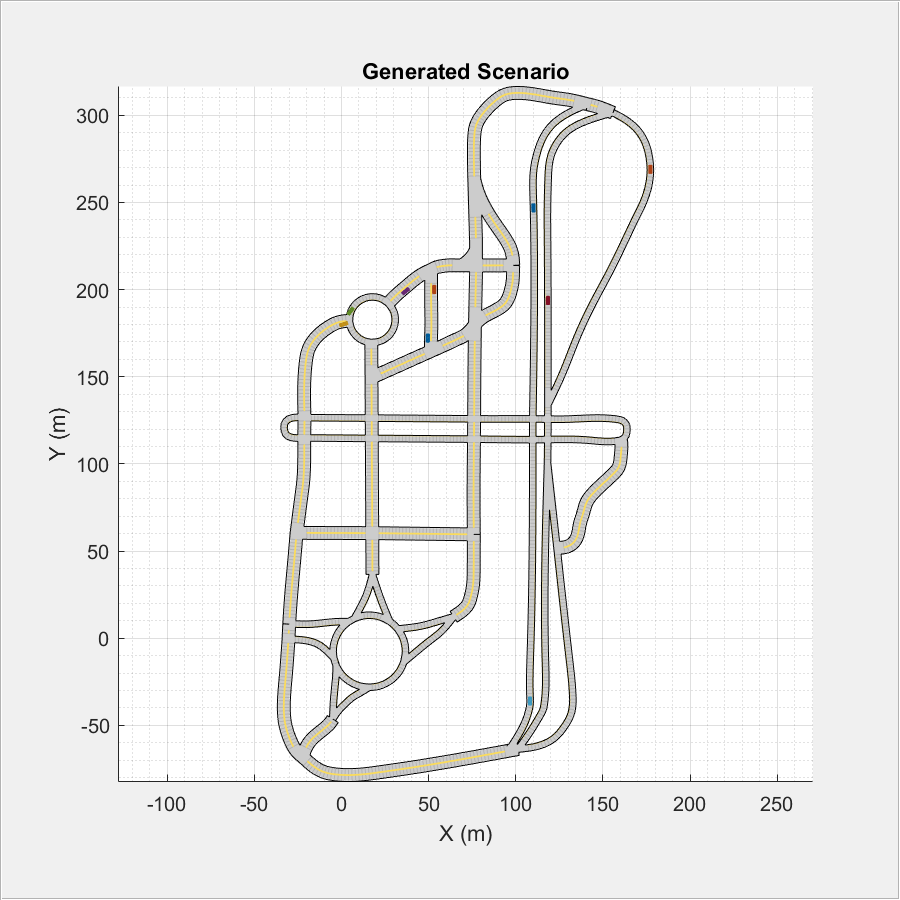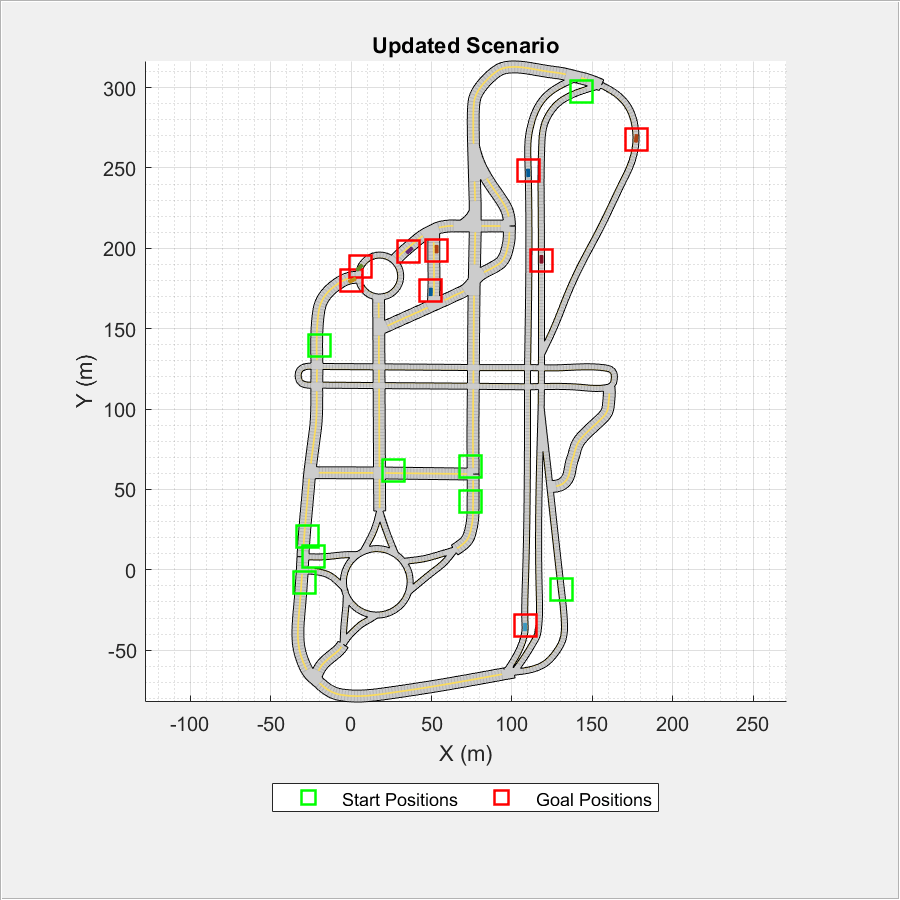# Automatic Scenario Generation

This example shows how to automate scenario generation by using a `drivingScenario` object. In this example, you will automate:

• Vehicle placements in a scenario by defining their start and goal positions

• Waypoint selection and trajectory generation for the vehicles to traverse from their start positions to goal positions.

• Speed adjustment such that the vehicles accelerate or decelerate to avoid colliding between other vehicles that travel in the same lane.

You can use this example to synthesize a number of random scenarios for testing driving algorithms.

### Introduction

The `drivingScenario` object and the Driving Scenario Designer app in Automated Driving Toolbox™ are efficient tools for generating synthetic driving scenarios. You can create a road network or import a road network from OpenDRIVE®, HERE HD Live Map, and OpenStreetMap®. Then, you can add actors or vehicles to the road network and define their trajectories to synthesize a driving scenario. The waypoints required for generating the trajectories must be selected carefully such that the trajectories of the vehicles lie within the road network and the vehicles does not collide as they travel along their trajectories. Defining such vehicle placements and trajectories often requires multiple trials and is time consuming if you have large road networks and many vehicles to configure.

This example provides helper functions and demonstrates the steps to automate vehicle placements and trajectory generation by using the `drivingScenario` object. You can also export the generated scenario to the Driving Scenario Designer app. The rest of the example demonstrates these steps involved in automating scenario generation.

1. Import road network - Import OpenStreetMap® road network into a driving scenario object by using the helper function `helperOSMimport`.

2. Define start and goal positions - Specify regions of interest (ROIs) in the road network to select the start and goal positions for vehicles by using the helper function `helperSamplePositions`.

3. Generate vehicle trajectories - Generate waypoints and trajectories by using the helper function `helperGenerateWaypoints` and the `trajectory` function`.`

4. Modify speed profiles to avoid collision - Modify the speed profiles of the vehicles in the scenario by using the Simulink® model `CollisionFreeSpeedManipulator`. The model checks the speed profile of each vehicle and prevents them from colliding with each other as they travel along their trajectories. The output from the model is an updated scenario that is free from collision between vehicles. You can convert the output from the `CollisionFreeSpeedManipulator` Simulink model to a driving scenario object by using the helper function `helpergetCFSMScenario`.

5. Simulate and visualize generated scenario - Simulate and display the automatically generated scenario by using the `plot` function. You can also read and simulate the scenario by using the Driving Scenario Designer app.

Specify the bounding box coordinates to import the MCity test facility map from `openstreetmap.org` by using the `helperOSMImport` function. The function returns a driving scenario object that contains the road network from the imported map data. You can also use the `roadNetwork` function to import a road network from OpenDRIVE®, HERE HD Live Map, or OpenStreetMap® files.

```% Import the road network of MCity minLat = 42.2990; maxLat = 42.3027; minLon = -83.6996; maxLon = -83.6965; bbox = [minLat maxLat;minLon maxLon]; scenario = helperOSMImport(bbox); % Create another scenario object for plotting purposes scenario_in = helperOSMImport(bbox);```

Display the MCity road network by using the `plot` function.

```figure plot(scenario_in) title("Imported Road Network") xlim([-50 190]) ylim([-85 330])```### Define Start and Goal Positions

To create a driving scenario, you must first define specific points on the road network that can serve as start and goal positions for the vehicles in the scenario. Use the `helperSamplePositions` function to generate a random set of these points in the road network. You can use one or more of these name-value pair arguments of `helperSamplePositions` functions to configure the start and goal positions in different ways"

• Use '`Seed`' to specify the random generator settings to be used for generating random points. You can select any points in the generated set as start and goal positions.

• Use '`ROI`' to specify one or more ROIs in the road network within which you want to define the start and goal positions. The ROIs can be circular, rectangular, or a polygon region with any number of vertices. The value for ROI is an N-by-2 matrix specifying the spatial coordinates of a closed region. If this value is not specified, the function generates random points across the entire road network.

• Use '`Lanes`' to specify the lanes in which you want to define the start and goal positions. To select a single lane, specify the lane number as a scalar value. For multiple lanes, the value of '`Lanes`' must be a vector containing the desired lane numbers. If this value is not specified, the function selects the lanes randomly.

• Use '`LongitudinalDistance`' to set the longitudinal distance between two consecutive points. If this value is not specified, the function imposes at least 5 meters of distance between two consecutive points in the same lane. This implies that the longitudinal distance between two consecutive vehicles placed in the same lane is at least 5 meters.

During simulation, the vehicles spawn at the start points and then travel to reach the goal points.

#### 1. Select Start Positions

Generate 10 random points to use as potential start positions. Specify a flag for setting the random number generator. Set the value for `setSeed` to 1 to specify the seed for random number generator. Pass the random generator settings as input to the `helperSamplePositions` function by using the '`Seed`' name-value pair argument.

The `helperSamplePositions` function outputs the 3-D spatial coordinates of the randomly selected points in the imported road network. The function also outputs the yaw angles relative to the selected points. The yaw angle obtained relative to a point defines the orientation for the vehicle to be placed at that point.

```numPoints = 10; setSeed = 2; rng(setSeed); s = rng; [points,yaw] = helperSamplePositions(scenario,numPoints,"Seed",s);```

Specify the number of vehicles to be placed in the scenario as 3. Select any three points in the generated set as the start positions for the vehicles.

```numVehicles = 3; startSet1 = [points(2,:);points(4,:);points(7,:)]; yaw1 = [yaw(2);yaw(4);yaw(7)]; mesh = driving.scenario.carMesh;```

Place vehicles in the selected points by using the `vehicle` function.

```for idx = 1 : numVehicles vehicle(scenario,Position=startSet1(idx,:),Yaw=yaw1(idx),ClassID=1,Mesh=mesh); end```

Generate another set of points by defining the ROIs. Compute the coordinates to specify a circular ROI.

```xCor = 0; yCor = 0; radius = 50; theta = 0: pi/10: 2*pi; roiCircular(:,1) = xCor+radius*cos(theta); roiCircular(:,2) = yCor+radius*sin(theta);```

Specify the number of points to be generated within the ROI and the number of vehicles to place within the ROI as 3. Select all the points within the circular ROI as the start positions for the vehicles.

```numPoints = 3; [startSet2,yaw2] = helperSamplePositions(scenario,numPoints,ROI=roiCircular); for idx = 1 : size(startSet2,1) vehicle(scenario,Position=startSet2(idx,:),Yaw=yaw2(idx),ClassID=1,Mesh=mesh); end```

Specify the coordinates for a rectangular ROI. Set the number of points to be generated within the ROI and the number of vehicles to place within the ROI as 3. Set the longitudinal distance between two consecutive points in the same lane to 30 meters. If the ROI is not large enough to accommodate the specified number of points at the specified longitudinal distance, then the `helperSamplePositions` function returns only those number of points that can be accommodated within the ROI. To get the desired number of points, you must either reduce the longitudinal distance or increase the area of the ROI.

```roiRectangular = [0 0;100 100]; numPoints = 3; [startSet3,yaw3] = helperSamplePositions(scenario,numPoints,ROI=roiRectangular,LongitudinalDistance=30);```

Place vehicles at the selected points by using the `vehicle` function.

```for idx = 1 : size(startSet3,1) vehicle(scenario,Position=startSet3(idx,:),Yaw=yaw3(idx),ClassID=1,Mesh=mesh); end```

Plot the generated sample points and the ROIs.

```figScene = figure(Name="AutomaticScenarioGeneration"); set(figScene,Position=[0,0,900,500]); hPanel1 = uipanel(figScene,Position=[0 0 0.5 1]); hPlot1 = axes(hPanel1); plot(scenario_in,Parent=hPlot1,Meshes="on"); title("Points for Selecting Start Positions") hold on plot(points(:,1),points(:,2),"ro",MarkerSize=5,MarkerFaceColor="r"); plot(roiCircular(:,1),roiCircular(:,2),LineWidth=1.2,Color="k"); plot(startSet2(:,1),startSet2(:,2),"ko",MarkerSize=5,MarkerFaceColor="k"); plot([roiRectangular(1,1);roiRectangular(1,1);roiRectangular(2,1);roiRectangular(2,1);roiRectangular(1,1)], ... [roiRectangular(1,2);roiRectangular(2,2);roiRectangular(2,2);roiRectangular(1,2);roiRectangular(1,2)], ... LineWidth=1.2,Color="b"); plot(startSet3(:,1),startSet3(:,2),"bo",MarkerSize=5,MarkerFaceColor="b"); xlim([-50 190]) ylim([-85 330]) hold off```

Display the start positions and the vehicles in the scenario.

• The 3 start positions in red were selected from the 10 random points defined throughout the scenario.

• The 3 start positions in black were selected from the 3 random points defined in the circular ROI.

• The 3 start positions in blue were selected from the 3 random points defined in the rectangular ROI.

```hPanel2 = uipanel(figScene,Position=[0.5 0 0.5 1]); hPlot2 = axes(hPanel2); plot(scenario,Parent=hPlot2,Meshes="on"); title("Start Positions and Vehicle Placement") hold on plot(startSet1(:,1),startSet1(:,2),"rs",MarkerSize=15,LineWidth=1.2); plot(startSet2(:,1),startSet2(:,2),"ks",MarkerSize=15,LineWidth=1.2); plot(startSet3(:,1),startSet3(:,2),"bs",MarkerSize=15,LineWidth=1.2); xlim([-50 190]) ylim([-85 330]) hold off```Merge all the start positions into a single matrix. The number of start positions implies the total number of vehicles in the driving scenario.

`startPositions = [startSet1;startSet2;startSet3];`

#### 2. Inspect Scenario Object

Display the scenario object and inspect its properties. The `Actors` property of the `scenario` object is a 1-by-9 array that stores information about the 9 vehicles that are added to the driving scenario. Access the details of each vehicle in `Actors` property by using dot indexing. Display the details about the first vehicle in the driving scenario. The `Position` property contains the start position of the vehicle.

`scenario`
```scenario = drivingScenario with properties: SampleTime: 0.0100 StopTime: Inf SimulationTime: 0 IsRunning: 1 Actors: [1×9 driving.scenario.Vehicle] Barriers: [0×0 driving.scenario.Barrier] ParkingLots: [0×0 driving.scenario.ParkingLot] ```
`scenario.Actors(1)`
```ans = Vehicle with properties: FrontOverhang: 0.9000 RearOverhang: 1 Wheelbase: 2.8000 EntryTime: 0 ExitTime: Inf ActorID: 1 ClassID: 1 Name: "" PlotColor: [0 0.4470 0.7410] Position: [130.7903 -12.2335 -2.0759e-04] Velocity: [0 0 0] Yaw: 96.6114 Pitch: 0 Roll: 0 AngularVelocity: [0 0 0] Length: 4.7000 Width: 1.8000 Height: 1.4000 Mesh: [1×1 extendedObjectMesh] RCSPattern: [2×2 double] RCSAzimuthAngles: [-180 180] RCSElevationAngles: [-90 90] ```

#### 3. Select Goal Positions

Generate the goal positions for the vehicles in the scenario by using the `helperSamplePositions` function. The total number of goal positions must be the same as the total number of start positions.

`numGoalPositions = length(startPositions)`
```numGoalPositions = 9 ```

Specify the coordinates for a polygon ROI and find 5 random points within the polygon ROI. Select these points as the goal positions for the first 5 vehicles in the scenario.

```roiPolygon = [-50 170;30 250;72 170;-50 170]; numPoints1 = 5; goalSet1 = helperSamplePositions(scenario,numPoints1,ROI=roiPolygon);```

Generate the remaining set of goal positions in such a way that they all lie in a specific lane. Use the '`Lanes`' name-value pair argument to specify the lane number for goal positions.

```numPoints2 = 4; goalSet2 = helperSamplePositions(scenario,numPoints2,Lanes=1);```Display the scenario and the selected goal positions.

• The 5 points in red show the goal positions defined in the polygon ROI.

• The 4 points in blue show the goal positions defined across the entire scenario.

```figure plot(scenario,Meshes="on"); title("Goal Positions") hold on plot(roiPolygon(:,1), roiPolygon(:,2), LineWidth=1.2, Color="r") plot(goalSet1(:,1), goalSet1(:,2), "ro", MarkerSize=5, MarkerFaceColor="r") plot(goalSet2(:,1), goalSet2(:,2), "bo", MarkerSize=5, MarkerFaceColor="b") xlim([-50 190]) ylim([-85 310]) hold off```Merge all the goal positions into a single matrix.

`goalPositions = [goalSet1;goalSet2];`

Display the start positions and the goal positions with respect to each vehicle in the scenario.

```vehicleNum = 1:length(startPositions); table(vehicleNum(:),startPositions,goalPositions,VariableNames={'Vehicle','Start positions','Goal positions'})```
```ans=9×3 table Vehicle Start positions Goal positions _______ _____________________________________ _______________________________________ 1 130.79 -12.233 -0.00020759 49.544 173.51 0.0009993 2 -19.606 139.45 0.00065289 53.19 198.74 0.00070198 3 143.37 297.8 -0.00188 -0.019449 179.76 0.00058451 4 -23.372 8.0685 -0.00014976 35.769 198.22 0.00062871 5 -27.346 20.561 -4.1238e-05 6.0029 188.74 0.00056541 6 -29.047 -7.817 -0.00053606 108.15 -34.789 -0.00049719 7 26.393 62.042 0.00095438 118.42 192.5 0.00054377 8 73.989 42.717 0.00094018 110.09 248.17 -0.00032446 9 73.996 64.436 0.0011401 177.2 267.68 -0.0015615 ```

### Generate Vehicle Trajectories

Use the `helperGenerateWaypoints` function to compute waypoints that connect the start and goal positions. The function returns a structure array that contains the road centers, computed waypoints, and yaw angle for each vehicle in the scenario. Read the vehicle information from the `scenario` object and specify random speed values for each vehicle. Use the `trajectory` function to generate the trajectories for each vehicle by using the computed waypoints and random speed values.

```info = helperGenerateWaypoints(scenario,startPositions,goalPositions); for indx = 1:length(startPositions) vehicleData = scenario.Actors(indx); speed = randi([10,25],1,1); waypts = info(indx).waypoints; trajectory(vehicleData,waypts,speed); end```

Set the stop time for the scenario.

`scenario.StopTime = 50;`

Create a custom figure and display the simulated driving scenario.

```close all; figScene = figure; set(figScene,Position=[0,0,600,600]); movegui(figScene,"center"); hPanel = uipanel(figScene,Position=[0 0 1 1]); hPlot = axes(hPanel); plot(scenario,Parent=hPlot,Meshes="on"); title("Generated Scenario") while advance(scenario) end```In the generated scenario, all the vehicles traverse along their trajectories at a particular speed to reach their goal positions. You can also observe collision between two actors as they traverse along their trajectories. While you synthesize a scenario for testing driving algorithms, it is important that the vehicles in the scenario do not collide. To prevent collision, you must adjust the velocity of the vehicles so that they do not collide with each other while traveling along their paths.

### Modify Speed Profile to Avoid Collision

Use the MATLAB® System object™ `CollisionFreeTrajectory` to correct the velocity of the vehicles such that they do not collide as they traverse along their trajectories. The model uses nonlinear time scaling to reactively accelerate or decelerate a vehicle without altering its trajectory .

```collisionFreeObj = helperCollisionFreeTrajectory(Scene=scenario); out = cell(numel(0:0.025:50),1); scenario.SampleTime = 0.025; index = 1; for time = 0:0.025:50 out{index} = collisionFreeObj(time); index = index+1; end```

### Simulate and Visualize Generated Scenario

Use the `helpergetCFSMScenario` function to convert the output from `CollisionFreeTrajectory` to a driving scenario object. Simulate and display the driving scenario. You can see that the vehicles travel along the specified trajectories to reach their goal positions.

```newScenario = helperCreateCFSMScenario(out,scenario); close all; figScene = figure; set(figScene,Position=[0,0,600,600]); movegui(figScene,"center"); hPanel = uipanel(figScene,Position=[0 0 1 1]); hPlot = axes(hPanel); plot(newScenario,Parent=hPlot,Meshes="on"); title("Updated Scenario") hold on h1 = plot(goalPositions(:,1),goalPositions(:,2),"rs",MarkerSize=15,LineWidth=1.2); h2 = plot(startPositions(:,1),startPositions(:,2),"gs",MarkerSize=15,LineWidth=1.2); legend([h2 h1],{"Start Positions";"Goal Positions"},Location="southoutside",Orientation="horizontal") hold off while advance(newScenario) end```You can also export the scenario to Driving Scenario Designer app and run the simulation.

`drivingScenarioDesigner(newScenario)`### Tips To Avoid Collisions

The MATLAB System object `CollisionFreeTrajectory` adjusts only the speed profile of the active vehicles. Once the vehicle reaches the goal position and becomes inactive in the scenario, it is not considered for checking collisions. If you want to generate a driving scenario with non-colliding vehicles, select points at less proximities and in different lanes as the start and the goal positions. If there is another vehicle whose goal position is close to the non-active vehicle and trajectory is same as the non-active vehicle's trajectory then there will be collision between these vehicles. Similarly, collision occurs when two vehicles travelling in same or different lanes come in close proximity at the road intersections. Also, the chances for collision is more if two or more goal positions lie in the same lane.

1.2.Singh, Arun Kumar, and K. Madhava Krishna. “Reactive Collision Avoidance for Multiple Robots by Non Linear Time Scaling.” In 52nd IEEE Conference on Decision and Control, 952–58. Firenze: IEEE, 2013. https://doi.org/10.1109/CDC.2013.6760005.